Home > AC > Chapter 11 > Lesson 11.1.1 > Problem11-10

11-10.
1. Solve the following inequalities for x. Homework Help ✎

1. 4x − 1 > 7

2. 3 − 2x < x + 6

3. 2(x − 5) < 8

4.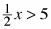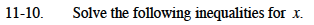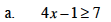Solve as an equation to find the boundary point.
Check points in each region to determine which region is the solution.

x ≥ 2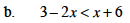Use the same method as part (a).

x > -1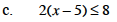Distribute before solving.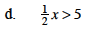Multiply the whole inequality by 2 to cancel out the fraction.